﻿ The Effect of <i>Think Talk Write</i> Learning Model with Batak Toba Culture Context toward Students’ Mathematical Communication Skill of SMP Swasta Parulian 2 MedanPublications are Open
Access in this journal
Article Versions
Export Article
• Normal Style
• MLA Style
• APA Style
• Chicago Style
Research Article
Open Access Peer-reviewed

### The Effect of Think Talk Write Learning Model with Batak Toba Culture Context toward Students’ Mathematical Communication Skill of SMP Swasta Parulian 2 Medan

Syuhada Sitompul , Edi Syahputra, Kms. M. Amin Fauzi
American Journal of Educational Research. 2019, 7(2), 183-188. DOI: 10.12691/education-7-2-12
Received December 11, 2018; Revised January 18, 2019; Accepted February 23, 2019

### Abstract

The aim of this study are to, know the effect of Think Talk Write learning model with Batak Toba culture context toward student mathematical communication skill significantly, know the effect of beginning mathematical skill (High, Medium, Low) toward student mathematical communication skill, know whether there is interaction between (TTW, Conventional) learning model and beginning mathematical skill (High, Medium, Low) toward student mathematical communication skill significantly. The data analysis is done by two way of analysis variance (ANAVA). The result of study shows, there is effect of Think Talk Write learning model with Batak Toba culture context toward student mathematical communication skill significantly, there is effect of mathematical beginning skill (High, Medium, Low) toward student mathematical communication skill, there is no interaction between (TTW, Conventional) learning model and mathematical beginning skill (High, Medium, Low) toward student mathematical communication skill significantly. The test instrument of this study is student mathematical communication skill.

### 1. Introduction

Education is the most precious human resources investation for human life in the world. Indonesia as one of country in the world has placed education as important and main thing. It can be proved on preamble of the 1945 law on the fourth line which emphasize that one of Indonesia purpose is enrich the nation’s life. The purpose can be achieved with effective and efficient education and learning, both formal and informal. The learning process is the main of formal education process in the school where there is interaction of variety component such as teacher, student and learning material in it. One of the most important characteristic in effective learning process is teachers’ skill cooperate with students also skill in organizing systematic study experience. In this case, the teacher should be able understand the students’ condition and organize study experience given by the students.

One of aspect which is emphasized in curriculum and NCTM is improve student mathematic communication skill. Basically, mathematic communication skill is the purpose and achieved study result in learning in every level of math education. Therefore, mathematic learning should be main lesson in order to achieve mathematic communication skill, make students master mathematic well and make student get achievement optimally. Based on the results of the study 1 states that mathematical communication skills need to be mastered by students, because mathematics is not just a tool to think, a tool to find patterns, solve problems and draw conclusions. To develop communication ability, the sender can tell in various language include mathematical language. If the students have communication skill, it will make students having deep mathematical understanding about mathematical concepts.

The cause of low capability of student mathematic communication skill is the students are not capable in communicating the mean of question given. It because the previous learning only explain the steps of counting without help students to express idea in written and spoken form. Besides, the students are still rigid with numbers so that when the students are given questions about story question or in symbol form the students are not capable in finishing the question. Therefore, in this case the students mathematic communication skill still extremely improved or in other words, students mathematic communication skill still extremely low. According 2 research stating that student mathematical communication is still very low. The students often face difficulties of learning math because the teacher only focus on counting and memorize formula which makes students less of achievement in learning. The students are not given chance to try developing their own skill. In line with the study 3 basically stated that from the results of student answers it was found that only 4 students solved the problem correctly from 15 students who took the test. That is, there were only 26.67% of students who could solve the problem correctly, and 73.33% of the other students answered incorrectly. This shows that students' mathematical communication skills are still low.

4 explains that there are two importance of why communication in mathematical need to be develop for students. First (mathematical as language), which means mathematic is not only (a tool to aid thinking), a tool to find formula, solve problem, make conclusion but also mathematic is as a tool to communicate various idea, or opinion, clearly, correctly and right. Second (mathematic learning as society activity), which means mathematic as social activity in mathematic learning and mathematic also as interaction tool between students and students, interaction tool between teacher and students. It is supported with 5 opinion that the importance of communication skill for the students in mathematic learning are: 1) connect real things, picture and diagram into mathematic idea; 2) explain idea, situation and mathematic relation verbally and non verbally with real thing, picture, graph and algebra; 3) express daily experience into mathematic language or symbol; 4) listening, discussing, and writing about mathematic; 5) reading with written mathematic understanding and representation; 6) make conjecture arrange argument, pattern the definition and generalization and; 7) explain and make question about previous mathematic learning..

The important thing that teacher need to pay attention in learning in the class beside student mathematical communication skill is student mathematical beginning skill. The student mathematic beginning skill is the skill which is possessed by students before mathematic learning process is done in the class. It is caused because the learning material is arranged structurally so if a student are difficult to understand the beginning material, the students will be difficult to learn next material automatically. The beginning skill which is possessed by the student vary each other. It can be seen from student mastery level which means the skill can differ between students which possess high, medium and low skill. In fact, the teachers seldom to pay attention mathematic beginning ability which possess by students. 6 said that teachers need to know knowledge about student mathematic beginning skill to decide teaching strategy even to give question or problem to students. Based on understanding about student mathematic beginning skill, the teacher can help students to accelerate the learning process and minimize students’ difficulties in understanding learning material taught by teachers. It shows that student mathematic beginning skill will affect learning either taught by learning model or conventional teaching. The mathematic beginning skill surely will affect improvement of student mathematic communication skill.

One cause of the low capability of student mathematic communication skill which makes students are difficult in understanding mathematic learning is the learning model used by the teachers. The teacher must create learning situation which is able to explore students’ skill in solving problem. Based on results of study 7 it is also expected that teachers can design the existing learning from an early age with the environment in the educational environment to achieve the goals set goals. One of learning which is considered able to develop student mathematic communication skill is a learning model which is interesting and understand abstract mathematic concepts. One of method which make student be active in learning process is cooperative learning model. Cooperative learning model is learning model which involve students to interact, cooperate with friends and can give chance to students to construct ideas. The learning model is assumed able to attract students’ interest to learn mathematic then will affect on students mathematic communication ability and change students view into positive view about mathematic. The learning model explained above is called Think Talk Write learning model.

Think Talk Write learning model is learning model which can grow communication skill and there are steps in doing the learning model namely think, talk and write. Think step, can be seen when students read a text or mathematic story then note what they have read. In noting, the students translate what they read into their own language. Note can increase student knowledge and improve thinking and writing skill. Talk step, the students use language to give idea to their friends, build theory together, share strategy and solution and make definition. Write step, this step means construct idea through writing. Writing in mathematic help realizing one of purpose namely students understanding about what material they learnt.

Based on 8 result analysis said that student mathematic communication skill taught by TTW learning model is significantly higher than taught by conventional teaching. The mean of mathematic communication test in experiment class is 83.84 and the mean in control class is 48 for man and woman students. The same with 9 research concluded that there is skill improvement of mathematic concept understanding by applying Think Talk Write learning model with mean score is 79.07 and mathematic communication skill with mean 80.37.

The application of Think Talk Write learning model will be more effective if there is culture integration into learning at school. The learning will be more useful in local culture and learning process by applying culture system and culture values where the students live. Integration with Batak Toba culture context into mathematic learning can give chance for teachers to improve mathematic communication skill and student social skill also introduce variety of Batak Toba culture context which near with children environment so that the preservation is kept and the opportunity to develop it is opened in school environment. The reason in choosing Batak Toba culture caused by the majority in SMP Swasta Parulian 2 Medan is Batak Toba.

### 2. Theoretical Review

Mathematic communication skill define as skill to state, to demonstrate and to define mathematic idea from a contextual problem in description form into mathematic model (picture, graph, diagram, table and equality) or otherwise. The students can have mathematic communication skill when they learn in pairs, when they explain about logarithm to solve problem, when they construct and explain graph representation toward real phenomenon or when they give a conjecture about geometric pictures 10 NCTM.

Mathematic communication skill in this research is: 1) express, demonstrate, and draw mathematic ideas into mathematic picture, table, graph or other form; 2) state a situation, picture, diagram or real thing into mathematic language, symbol, idea, or model; 3) analyze, evaluate, and give question toward an information given.

### 3. Methods

The research is categorized into quasi experimental research which is done in SMP Swasta Parulian 2 Medan. The population of research is all students of seventh grade students with total class is 5 classes and total student is 200 students. The sample of research is VII-1 and VII-2. The experiment class is VII-1 and VII-2 as control class. The design which is used in this research is Non Equivalent Control Group Design as Table 1 follows:

The technique of collecting data in this research using test to measure student mathematical communication skill. The data processing in hypothesis test started by testing statistic precondition which is needed namely normality test and variance homogeneity test, then the researcher do two way variance analysis (ANAVA). All statistic calculation is done by Microsoft Excel computer program. Statistic model of this research 11 is: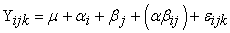with: i = 1, 2; j = 1, 2, 3; k = 1, 2, ...,n.

### 4. Discussion

Mathematic beginning skill test given to know the equality of experiment class and control class mean and to group student based on mathematic beginning skill namely high, medium and low. Here are the mean calculation summary and standard deviation of mathematic beginning skill served in Table 2 as follows:

The Table 2 above shows that mean and deviation standard for student in experiment class with high mathematical beginning skill category is 79,00 and 3,34, medium mathematical beginning skill is 59,67 and 12,70, low mathematical beginning skill is 27,50 and 5,83 meanwhile in control class students score who includes in high category of mathematical beginning skill is 78,86 and 3,02, medium mathematical beginning skill is 57,08 and 10,28, low mathematical beginning skill is 26,86 and 5,01.

Based on Table 3 above gained that D0 score for experiment class is 0,100 and for control class is 0,094. D0 score for both classes is less than Dtable, which means the data include to normal distributed for experiment class and control class, and Table 4 above gained that Fcalculate < Ftable, namely 1,342 < 1,704 which mean variance of data group is homogen. It shows that both of data group for experiment class and control class have homogen data variance.

Based on Table 5 above seen that mean score of student mathematic communication skill taught by Think Talk Write learning model with Batak Toba culture context is 74,20 while mean score of student mathematic communication skill taught by conventional teaching is 42,59. Mean score of student mathematic communication skill in experiment class is higher than mean score of student mathematic communication skill in control class.

From the data of mathematical communication skill test score, then the researcher analyze to know the difference of student mathematical communication skill which is taught by Think Talk Write learning model with Batak Toba culture context and which is taught by conventional teaching based mathematical beginning skill of students. All result of mathematical communication skill based on students mathematical beginning skill served on Table 6 as follows:

Table 6 shows that mean and deviation standard of student mathematical communication ability on experiment class with high mathematical beginning skill is 80,88 and 17,28, medium mathematical beginning skill is 73,75 and 14,72, low mathematical beginning skill is 69,00 and 14,16. While, student mathematical communication skill on control class with high mathematic beginning skill is 50,00 and 16,58, medium mathematical beginning skill is 43,88 and 11,80, low mathematical beginning skill is 30,29 and 4,50.

From data of mathematic communication skill test score based on mathematical beginning skill, then the researcher analyze to know difference of student mathematical communication skill which is taught by Think Talk Write learning model with Batak Toba culture context and which is taught by conventional teaching based on mathematic communication indicator. All result of mathematic communication skill analysis based on mathematic communication indicator served on Table 7 as follows:

Table 7 shows that mean of student mathematic communication skill on experiment class with indicator 1 is 122,14, indicator 2 is 110,50 and indicator 3 is 121,67. While, student mathematic communication skill on control class with indicator 1 is 78,00, indicator 2 is 49,75 and indicator 3 is 69,67.

Based on Table 8 above seen that D0 score is 0,098 and 0,156 for experiment class and control class. D0 score for both class is lower than Dtable so that the data included to normal distributed for Think Talk Write learning model class with Batak Toba culture context and for conventional teaching class, and Table 9 above seen that score of Fcalculate < Ftable is 1,344 < 1,704 so that the sample comes from homogen variance group of data. It shows that both data group of experiment class and control class have homogen variance data.

Based on Table 10 gained tht F0 score (A) is 107,999, if F0 (A) is higher than Ftable (107,999 > 3,970) which can be concluded that the researcher has enough prove to reject H0. There is effect of learning model toward student mathematic communication skill. Then, gained F0 score (B) is 4,889, if F0 (B) is higher than Ftable (4,889 > 3,120) which can be concluded that the researcher has enough prove to reject H0 means there is effect of mathematical beginning skill toward student mathematic communication skill.

First Hypothesis

Proposed hypothesis is there is significant effect of Think Talk Write learning model with Batak Toba culture context toward student mathematic communication skill.

Statistic Hypothesis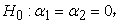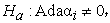with: i = 1, 2; j = 1, 2, 3

Based on Table 10 results analysis show that the F value is 107,999 > 3.970 and (sig) value 0,000 < 0,05, so that H0 is rejected and Ha is accepted. Then it can be concluded that there is significant effect of Think Talk Write learning model with Batak Toba culture context toward student mathematic communication skill.

To see how many percent the effect of Think Talk Write learning model with Batak Toba culture context toward mathematic communication skill, can be seen manually on Table 10 which shows effect of learning model toward student mathematic communication skill with score is 0,5722 (57,22%).

Second Hypothesis

The proposed hypothesis is there is effect of mathematic beginning skill (High, Medium, Low) toward student mathematic communication skill.

Statistic Hypothesis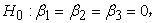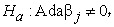with: i = 1, 2; j = 1, 2, 3

Based on Table 10 results analysis show that the F value is 4,889 > 3,120 and (sig) value 0,008 < 0,05, so that H0 is rejected and Ha is accepted. Then it can be concluded that there is effect of mathematic beginning skill (High, Medium, Low) toward student mathematic communication skill.

To see how many percent the effect of mathematic beginning skill (High, Medium, Low) toward mathematic communication skill can be seen manually on Table 10 which shows the effect of mathematic beginning skill (KAM) toward student mathematic communication skill with score is 0,0674 (6,74%).

Third Hypothesis

The proposed hypothesis is there is no significant interaction between learning model (TTW, Conventional) and mathematic beginning skill (High, Medium, Low) toward student mathematic communication skill.

Statistic Hypothesis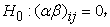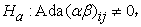with: i = 1, 2; j = 1, 2, 3

Based on Table 10 results analysis show that the F value is 0,677 < 3,120 and (sig) value 0,543 > 0,05, so that H0 is rejected and Ha is accepted. Then it can be concluded that there is no significant interaction between learning model (TTW, Conventional teaching) and mathematical beginning skill (High, Medium, Low) toward student mathematic communication skill.

To see how many percent the significant interaction between learning model (TTW, Conventional teaching) and mathematic beginning skill (High, Medium, Low) toward student mathematical communication skill can be seen manually on Table 10 which shows interaction of learning model and mathematical beginning skill (KAM) toward student mathematic communication skill with score is -0,0342 (-3,42%).

### 5. Conclusion

Based on result analysis about the effect of Think Talk Write learning model with Batak Toba culture context toward student mathematical communication skill, there are some conclusions as the answer of research question. They are, there is effect of Think Talk Write learning model with Batak Toba culture context toward student mathematical communication skill significantly. Based on result analysis, the Fcalculate (107,999) is higher than Ftable (3,970) which means that H0 is rejected and Ha is accepted; there is effect of mathematical beginning skill (High, Medium, Low) toward student mathematical communication skill. Based on result analysis, the Fcalculate (4,889) is higher than Ftable (3,120) which means that H0 is rejected and Ha is accepted; there is no significant interaction between learning model (TTW, Conventional) and mathematical beginning skill (High, Medium, Low) toward student mathematical communication skill. Based on result analysis, Fcalculate (0,677) is lower than Ftable (3,120) which means that H0 is accepted and Ha is rejected.

### 6. Suggestion

For teachers, the teachers can widen the using of Think Talk Write learning model in mathematic learning, not only in one variable linear equation but also in other mathematic learning material; the teachers are expected to add knowledge about innovative learning method and apply them in teaching and learning process; the teachers must create learning situation which function to express the summary of mathematic with the students language and way so that the students can be brave to argue more confidence and creative in studying mathematic.

For related institutions, Think Talk Write learning model with Batak Toba culture context by emphasizing student mathematical communication skill is still foreign for the teachers and students, therefore the school or related institutions need to socialize the learning method to improve student mathematic study result especially to improve student mathematic communication skill; Think Talk Write learning method with Batak Toba culture context can be used as one of learning alternative in improving student mathematic communication skill on one variable linear equation so that the Think Talk Write learning method can be input to be developed as an effective learning method on other mathematic material for the schools.

For the next researcher, if the next researcher would like to do research with the same method, the researcher must manage the time allocation to get the maximal result because the researcher will spend much time in applying Think Talk Write learning method with Batak Toba culture context; the research should be completed by doing research with other mathematic skill aspects namely understanding ability, reasoning ability, connection and mathematic representation more detailed and do the research not in the same school with the previous researcher; the next researcher should do research with different learning material; in doing the research, the next researcher should do the research based on indicator, especially on the second indicator about situation, picture, diagram, or real things into mathematic language, symbol or model.

### References

  Setiawati, D.; Syahputra, E.; & Rajagukguk, W. R. 2012. Perbedaan Peningkatan Kemampuan Pemecahan Masalah dan Komunikasi Matematik Siswa Antara Pendekatan Contextual Teaching and Learning dan Pembelajaran Konvensional Pada Siswa Kelas X SMK Negeri 1 Bireun. Jurnal Paradikma. Vol. 6, No.1 In article  Fahmi, A.; Syahputra, E.; & Rajagukguk, W. R. 2016. Peningkatan Kemampuan Penalaran dan Komunikasi Matematik Siswa Melalui Model Pembelajaran Berbasis Masalah Berbantuan Geogebra di Kelas VIII SMP Negeri 1 Samudera. Jurnal Paradikma. Vol.9, No.1. In article  Sibuea, M. F. L.; Asmin.; & Syahputra, E. 2015. Peningkatan Kemampuan Komunikasi dan Disposisi Matematis Siswa SMK Tamansiswa Sukadamai Kabupaten Asahan Melalui Model Pembelajaran Berbasis Masalah. Jurnal Paradikma. Vol.8, No.3. In article  Baroody, A. J. 1993. Problem Solving, Reasoning and Communicating, K-8 (Helping Children Think Mathematically). New York Mac Millan: Publishing Company. In article  Sumarmo, U. 2005. Alternatif Pembelajaran Matematika Dalam Menerapkan Kurikulum Berbasis Kompetensi (KBK). Bandung: UPI Bandung. In article  Achmad, N. 2011. Lima Kelemahan Mengajar Guru. http://www.pusatartikel.com/diakses. In article  Sapta, A.; Hamid, A.; & Syahputra, E. 2018. Assistance of Parents In The Learning At Home. Journal of Physics. Conference series, 1114(2018)012020. In article View Article  Chandra, S. R.; Fauzan. A.; & Helma. 2014. Pengaruh Model Pembelajaran Tipe Think Talk Write Dan Gender Terhadap Kemampuan Komunikasi Matematis Siswa Kelas VIII SMPN 12 Padang. Jurnal Pendidikan Matematika. Vol.3, No.1. In article  Armanto, D.; Sahyar.; & Anawati, S. 2016. Penerapan Model Pembelajaran Think-Talk-Write Untuk Meningkatkan Pemahaman Dan Komunikasi Matematik Siswa Kelas X SMA Al-azhar Medan. Jurnal Paradikma. Vol.9, No.1. In article  National Council of Teachers of Mathematics (NCTM). 1991. Professional Standard for Teaching Mathematics. Reston. VA: NCTM. In article  Syahputra, E. 2016. Statistika Terapan untuk Quasi dan Pure Experiment di Bidang Pendidikan, Biologi, Pertanian, Teknik, dll. Medan: Unimed Press. In article PubMed  PubMedThis work is licensed under a Creative Commons Attribution 4.0 International License. To view a copy of this license, visit http://creativecommons.org/licenses/by/4.0/